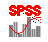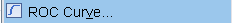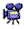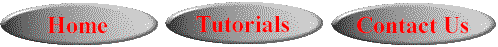# SPSS On-Line Training WorkshopHOME Table of Contents Data Editor Window Syntax  Editor WindowCarl Lee Felix Famoye About Us Chart Editor Window Output Window Overview of Data Analysis Manipulation of Data Analysis of Data Projects & Data Sets Integrate R into SPSS

# ROC CurveIn this Tutorial:

ROC Curve

Sensitivity

Specificity

Analysis of DataROC (Receiver Operating Characteristics)  Curve is useful for evaluating and comparing the performance of classification models where the response variable is binary. The following movie clip demonstrates how to create a ROC curve and make comparison of model performance.

In this on-line workshop, you will find many movie clips. Each movie clip will demonstrate some specific usage of SPSS.

ROC Curve: Useful for evaluating and comparing the performance of classification models where the response variable is binary (often labeled as Positive and Negative). This is a two-dimensional curve with the Y-axis, the sensitivity measure and  X-axis, (1-specificity). These sensitivity and (1-specificity) measures are computed based on a sequence of cut-off points to be applied to the model for predicting observations into Positive or Negative.

For example, a charity organization may be interested in classifying individuals into donor and non-donor based on a set of characteristics observed from the individual. There are different classification techniques that may be applied. The question is which technique gives the best classification. Various criteria may be used to evaluate the performance of a model. A common criterion is to select the model that results in the smallest misclassification rate. Another criterion is the use of ROC curve.

Sensitivity and Specificity that are used for constructing the ROC curve are described below.

A  classification modeling problem is to build a model for classifying each observation into two categories (Positive, Negative) of the binary response of interest. There are four possible consequences once the classification model is applied to a given observation:

(1) TP (True, Positive): The response is Positive, and the Prediction is also Positive.

(2) TN (True, Negative): The response is Negative, and the prediction is also Negative.

(3) FP (False, Positive): A negative response is falsely predicted as Positive. For example, a patient does not have cancer, but the model predicts the person has cancer. Then, the prediction is FP.

(4) FN (False Negative):  A positive response is falsely predicted as Negative.  For example, a patient does have cancer, but the model predicts the person has no cancer. Then, the prediction is FN.

Sensitivity is defined as the proportion of cases predicted as positive among all positive responses:  n(TP) / [(n(TP)+n(FN)]

Specificity is the proportion of cases predicted as negative among all negative responses. n(TN)/[n(TN)+n(FP)]

In terms of the Donor Vs. Non-donor example, sensitivity is the proportion of correctly predicted donors. It is the conditional probability of predicting a case as a donor given that the case is actually a donor. On the other hand, specificity is the proportion of correctly predicted non-donors. It is the conditional probability of predicting a case as a non-donor given that the case is indeed a non-donor.

The following movie clip demonstrates how to construct a ROC curve and how to use the curve to compare and select the 'best' model based on the ROC criterion.click here to watch ROC Curve

The data set for demonstrating ROC Curve is the Loan data set. See Data Set page for details. To construct the ROC curves for comparing the performances of different classification models, we will first need to build the classification models and save the estimated probability of classifying each case into the ‘positive’ category. The data used for this demonstration is a hypothetical data about approval of loans by a bank. The data set has 100 cases. The target variable, Loan = 1 if the bank approved a loan for the case, Loan = 0 if loan is not approved.This online SPSS Training Workshop is developed by Dr Carl Lee, Dr Felix Famoye , student assistants Barbara Shelden and Albert Brown , Department of Mathematics, Central Michigan  University. All rights reserved.